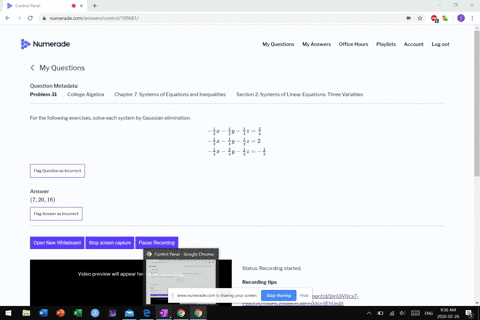Sign up for our free STEM online summer camps starting June 1st!View Summer Courses### For the following exercises, solve each system by…

05:04
MB

Need more help? Fill out this quick form to get professional live tutoring.

Get live tutoring
Problem 30

For the following exercises, solve each system by Gaussian elimination.
\begin{aligned} \frac{1}{2} x-\frac{1}{5} y+\frac{2}{5} z &=-\frac{13}{10} \\ \frac{1}{4} x-\frac{2}{5} y-\frac{1}{5} z &=-\frac{7}{20} \\-\frac{1}{2} x-\frac{3}{4} y-\frac{1}{2} z &=-\frac{5}{4} \end{aligned}

$\left(\frac{5}{22},-\frac{20}{11}, \frac{23}{11}\right)$

## Discussion

You must be signed in to discuss.

## Video Transcript

All right, Question 28. There's another galaxy elimination problem. This one they're not. All equations include all three variables. When I write those down, I like to write it down. So that so that the exes are all still lined up in the wiser. Lined up Caesar lined up. Um all right, so, uh, I'm seeing fractions in this. I could bust out those fractions. Also see that I could make all the exes same fairly easily. Um, bye. Turning them all into 12 x. Okay. So I'm gonna multiply this equation times for this equation. Times three. This equation by 12 against you tro ex and then half of whores to someone is to what minus for Izzy equals negative too. And then the second equation times three get 12 acts. There's no white term, so that's easy equals nine. And then find the last 1 12 x and then minus 18. Want the walls? Extensive fires. And so next thing to do is to subtract them. Was 12 months 12 0 So you gonna It's untracked. You start. So my 12 0 zero minus negative too, is to what, three minus negative for seven. See? So see, and that will equal nine minus *** to 11. Now, these two, I'm gonna subtract them. Um, I think Elsa tracked them down. Positive numbers again. So zero minus negative. 18. 18. And then there's no Z's not start three Z minus zero Z. It's trees E. And then nine minus negative. 30. All right. Um, all right. So I can multiply the top equation by nine. And so that I'll have 18. Why? And plus, um, 63 z equals 99. And then I took these two equations and also attract them. It's attractive, this direction right here to track them up. So 63 minus three is easy. It was 99 miles 39 60 c equals one. So and then to find why use, uh, this equation here? So, too, why plus seven z equals you, ever. And so I get minus seven from both sides to why he was four. So why he was too. Oh, it's nice. And so on. Then find the X er gracious. How about I like this equation? So I get four acts plus Z equals three. Um, so for X Nichols two equals so x true for what? One fuzzy